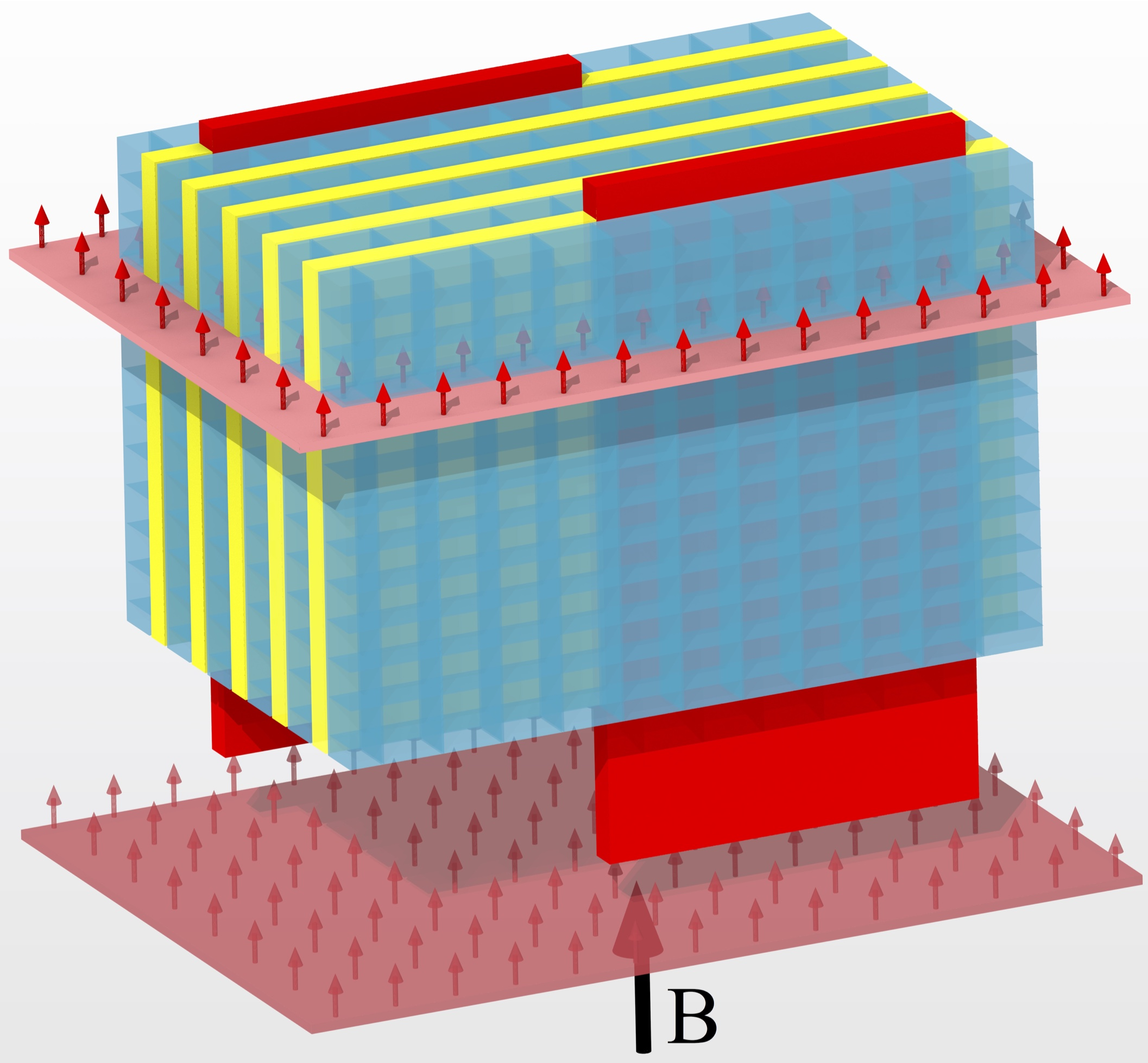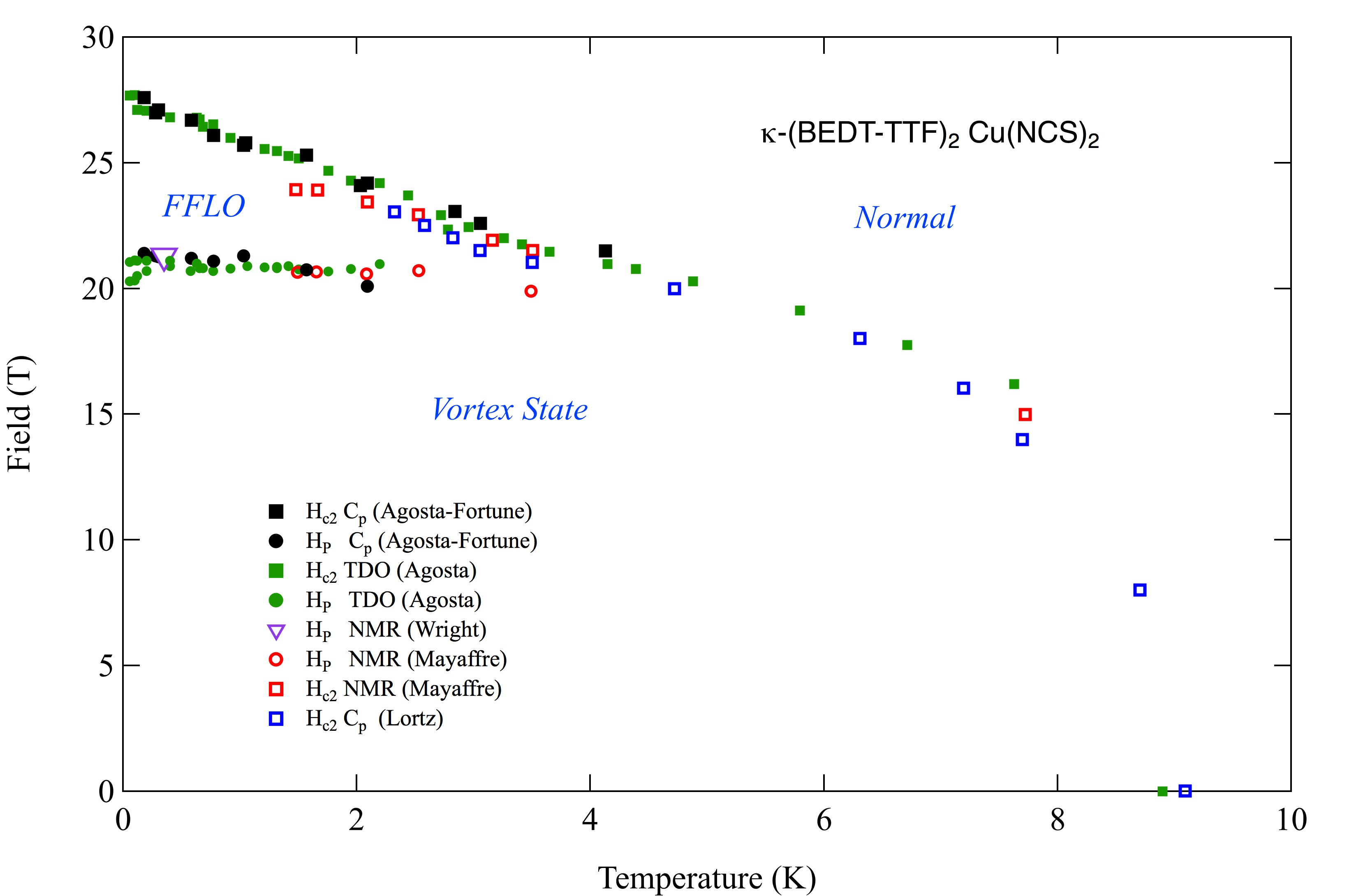## The Modulated superconducting Order Parameter - The FFLO State

#### Chuck Agosta - Clark University

Given the condition that superconductivity is destroyed by Pauli paramagnetism1,2 and that the superconductor is clean, as discussed on the previous page, it is energetically favorable for the superconducting order parameter to become modulated with areas of high and low concentrations of Cooper pairs in one, two, or three dimensions.34 One measure of the degree to which the superconductivity is destroyed by Pauli paramagnetism rather than vortices is the Maki parameter5 \begin{equation} \alpha_M = \sqrt{2}H^0_{orb}/H_P, \end{equation} where \begin{equation} H^0_{orb} = 0.7T_c\frac{dH_{c2}}{dT}|_{T_c},\label{eq:Horb} \end{equation} the orbital critical field at zero temperature,6 is the critical field as if there were no spin-paramagnetic pair breaking, just vortices. This formula was derived for 3D isotropic superconductors, and a for 2D materials the more complicated results in Klemm et al. 7 or Schneider et al.8 should be used, however for a simple comparison of the anisotropy between materials, the H$_{c2}$ slope near T$_c$ is valid. Therefore a large Maki parameter $\alpha_M$ suggests that the orbital pair breaking happens at a higher field than $H_P$, and Pauli paramagnetism will dominate, favoring inhomogeneous superconductivity. The other parameter r, defined above, is a measure of how clean the system is. It measures the ability for the material to support an extended wave function, necessary for the long range order of the FFLO state. In the ratio $r = \ell/\xi$, $\ell$ comes from the scattering time, which is related to the Dingle temperature, T$_D$, and the FermiA cartoon of a highly anisotropic superconductor with Josephson vortices and a modulated order parameter. velocity, $v_F = \sqrt{2E_F/m^*}$, where $E_F$ is the Fermi energy and $m^*$ is the effective mass. The Fermi energy, effective mass, and T$_D$, can all be measured via Shubnikov-de Haas (SdH) or de Haas-van Alphen oscillations. The other necessary parameter for finding r is $\xi$, which is found from the measurement of the superconducting critical field versus temperature and the formula $H_{c2} = \Phi_0/2\pi\mu_0\xi^2$, or estimated from the size of the superconducting energy gap, $\Delta$, and the formula $\xi = \hbar v_F/2\Delta$. Note that for the same Fermi energy, a higher effective mass results in a smaller $v_F$ and a smaller $\xi$, and hence a higher $H^0_{orb}$. For this reason heavy Fermion superconductors should favor the FFLO state. In general $H^0_{orb}$ comes from critical field measurements and $H_P$ can be found by analyzing specific heat measurements and determining the superconducting energy gap, as is described in detail on our page describing the Alpha Model. To the left is a cartoon of the FFLO state showing the nodes in the order parameter as horizontal planes where we estimate the spin-polarization to be $\approx 10\%$ at 25 T in the low temperature limit. In the diagram the black arrow labeled $\mathbf{B}$ represents the applied magnetic field, and the red arrows represent the net spin polarization. Although the diagram is schematic, all of the lengths are to scale; small boxes represent the unit cells of $\kappa$-(BEDT-TTF)$_2$Cu(NCS)$_2$, yellow slabs represent the least conducting layers of the crystal, and red rectangles represent Josephson core-less vortices at about the right distance apart in a 25 T field. Full height of the crystal is $\approx 20 nm$.

Below we show the temperature - magnetic field phase diagram of $\kappa$-(BEDT-TTF)$_2$Cu(NCS)$_2$ with the magnetic field orientated to favor paramagnetic limitng. The data comes from many different types of measurements, such as rf penetration(TDO), specific heat, and NMR. The specific heat measurements were particularly important as they established the the vortex FFLO phase line was first order, and the FFLO normal phase line as second order9.Votices in a layered super-conductor with a magnetic field applied perpendicular to the layers.
Assuming that we have found good values for $H_P$ , $H^0_{orb}$, $\ell$, and $\xi$, we should be able to predict which materials will exhibit a Clogston-Chandrasekhar limit or the FFLO state. Gruenberg and Gunther10 claim that if the Maki parameter &alpha > 1.6, a FFLO state is possible, or $H_{c2}$ could become a first-order transition below t = 0.55. $H_{c2}$ is normally a second-order transition in type II superconductors. Although Ref. 10 has certain assumptions that are only valid for BCS superconductors, this threshold is useful for the purpose of comparing different materials.

On the next page we will show how we calculate the Pauli paramagnetic limit, $H_P$, also known at the Clogston-Chandrasekhar limit that separates the vortex and FFLO phases, as well as the other parameters mentioned above. Or you could jump to a page containing a collection of phase diagrams of materials where we believe there is evidence for the FFLO state.

_______________________________________________

1 A. M. Clogston, "Upper Limit for the Critical Field in Hard Superconductors," Phys. Rev. Lett. 9, 266 (1962).
2 B. S. Chandrasekhar, "A Note on the Maximum Critical Field of High-Field Superconductors," Appl. Phys. Lett. 1, 7 (1962).
3 P. Fulde and R. A. Ferrell, "Superconductivity in a Strong Spin-Exchange Field," Phys. Rev. 135, A550 (1964).
4 A. I. Larkin and Yu. N. Ovchinnikov, "Inhomogeneous State of Superconductors," Zh. Eksp. Teor. Fiz. 47, 1136 (1964); or Sov. Phys. JETP 20, 762 (1965).
5 K. Maki, "Effect of Pauli Paramagnetism on Magnetic Properties of High-Field Superconductors," Phys. Rev. 148, 362 (1966).
6 Werthamer, N.R.; Helfand, E.; Hohenberg, P.C., "Temperature and Purity Dependence of the Superconducting Critical Field, $H_{c2}$. III. Electron Spin and Spin-Orbit Effects," Phys. Rev. 147, 295 (1966).
7 R. A. Klemm, A. Luther, and M. R. Beasley, "Theory of the upper critical field in layered superconductors," Phys. Rev. B 12, 877 (1975).
8 Schneider, T.; Schmidt, A., "Dimensional crossover in the upper critical field of layered superconductors," Phys. Rev. B 47, 5915 (1993).
9 Agosta, C.C. Fortune, N.A. Hannahs, S.T. Gu, S. Liang, L. Park, J.H. Schleuter, J.A. "Calorimetric Measurements of Magnetic-Field-Induced Inhomogeneous Superconductivity Above the Paramagnetic Limit," Phys. Rev. Lett. 118, 267001 (2017).
2222
Last updated 11 Dec 2018
Webmaster
cagosta@clarku.edu